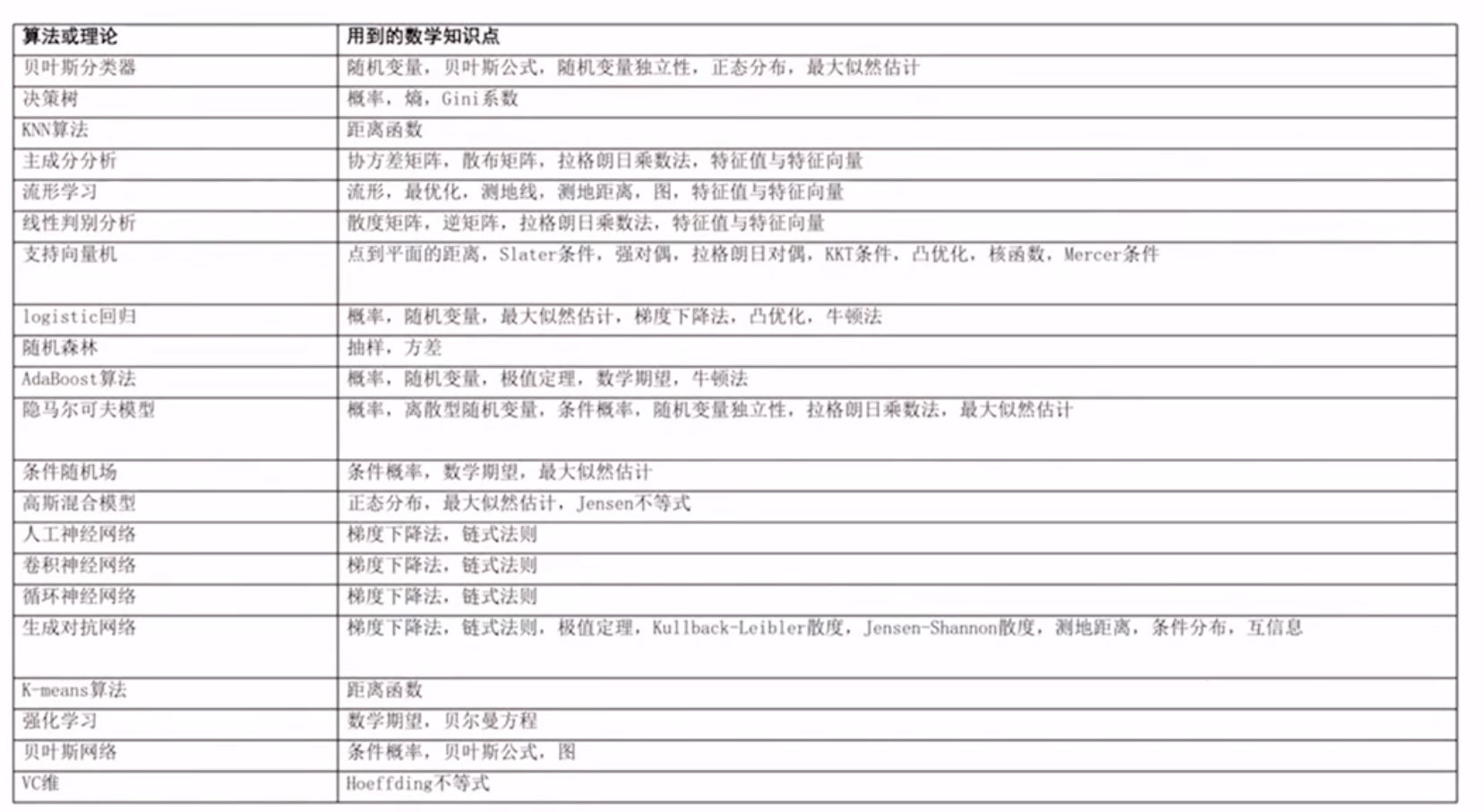【天池AI学习笔记】——AI数学基础

## 1. 机器学习需要涉及到哪些数学的知识

1. 微积分
2. 线性代数
3. 概率论
4. 最优化方法

Hessian矩阵## 2. 知识点

### 2.1 导数与高阶导数

1. 一阶导数反映的是函数斜率，而二阶导数反映的是斜率变化的快慢，表现在函数的图像上就是函数的凹凸性。

2. 函数的凹凸性：

f′′(x)>0,开口向上，函数为凹函数，f′′(x)<0,开口向下，函数为凸函数。

#例：
f(x) = x^2是凸函数，因为f′′(x)=2>0
f(x) = -x^2则是凹函数，因为f′′(x)=-2<0

3. 函数的拐点和驻点：

### 2.2 一元函数泰勒展开

1. 什么是泰勒展开式？就使用一个多项式函数来模拟一个可导的函数。具体来说就是：

1. 初始值相等，即： $g(x_{0}) = f(x_{0})$$x_{0}$为模拟的初始点。

2. n阶导数相等，即$g^{n}(x_{0}) = f^{n}(x_{0})$

$g(x) = a_{0} + a_{1}x + a_{2}x^{2} + ... + a_{n}x^{n}$

$g(0) = f(0) = a_{0}$，求出了 $a_{0}$

$g^{n}(0) = f^{n}(0)$

$g(x) = g(0) + \frac{f^{1}(0)}{1!}x + \frac{f^{2}(0)}{2!}x^{2} + \frac{f^{3}(0)}{3!}x^{3} + ... + \frac{f^{n}(0)}{n!}x^{n}$

$g(x) = g(x_{0}) + \frac{f^{1}(x_{0})}{1!}(x - x_{0}) + \frac{f^{2}(x_{0})}{2!}(x - x_{0})^{2} + \frac{f^{3}(x_{0})}{3!}(x - x_{0})^{3} + ... + \frac{f^{n}(x_{0})}{n!}(x - x_{0})^{n}$

$f(x) ≈ g(x) = g(x_{0}) + \frac{f^{1}(x_{0})}{1!}(x - x_{0}) + \frac{f^{2}(x_{0})}{2!}(x - x_{0})^{2} + \frac{f^{3}(x_{0})}{3!}(x - x_{0})^{3} + ... + \frac{f^{n}(x_{0})}{n!}(x - x_{0})^{n}$

$f(x) = g(x) = g(x_{0}) + \frac{f^{1}(x_{0})}{1!}(x - x_{0}) + \frac{f^{2}(x_{0})}{2!}(x - x_{0})^{2} + \frac{f^{3}(x_{0})}{3!}(x - x_{0})^{3} + ... + \frac{f^{n}(x_{0})}{n!}(x - x_{0})^{n} + ......$

-END-